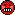## Excellent post! [BE/BA News]

Hi zizou,

congratulations for discovering this ‘goody’!

G. Confidence Interval Values for Unscaled Average Bioequivalence Analyses

❝ Sponsors should round off CI values to two decimal places. […]

❝ This change is equivalent to the change of acceptance interval from [80,125] to [79.995,125.005).

Yessir! Almost (see at then end).

❝ So we can correct acceptance limits in sample size calculations (just to be exact) and also edit the procedure (coded in statistical software) with comparison of exact values of CI with acceptance limits (round CI before comparison or equivalently change the acceptance limits).

❝ So it will be the same as according to EMA guideline now.Splendid.

❝ I was hoping for the change of EMA guideline to not round off CI values.

So did I. Everything else is bad science.
For my thinking about ‘nice numbers’ and rounding see there.

I think that the ‘experts’ of the FDA forgot (or worse: were not aware of) the origin of the limits, namely the clinically relevant difference $$\small{\Delta}$$:
$$\left\{\theta_1,\theta_2\right\}=\left\{100\,(1-\Delta),100/(1-\Delta)\right\}\tag{1}$$ We get ‘nice numbers’ of exactly $$\small{\left\{80\%,125\%\right\}}$$ only for $$\small{\Delta=20\%}$$. Since we analyze $$\small{\log_{e}}$$-transformed data, the limits are actually $$\small{\mp 0.22314355131421\ldots}$$ Not so nice.
Possibly the FDA is not aware of other jurisdictions where $$\small{\Delta=10\%}$$ is applicable for NTIDs or $$\small{\Delta=25\%}$$ for HVD(P)s. Then the upper limits are periodic ($$\small{\dot{1}11.11\%}$$ and $$\small{1\dot{3}3.33\%}$$).

❝ Now, I would write the acceptance interval [79.995,125.005) in the study protocols as these values are exact and no questions about TIE > 5 % can arise! ;DOh dear! See there. $$\small{125.005}$$ only if the software supports scientific rounding. Crappy software apply commercial rounding and then you would have to state $$\small{124.004\dot{9}}$$.

We can calculate the realized $$\small{\widehat\Delta_\textrm{r}}$$ from the limits: $$\widehat\Delta_\textrm{r}=\left\{\begin{array}{ll}\tag{2} 100\,(1-\theta_1/100)\\ 100\,(1-100/\theta_2) \end{array}\right.$$ Works as expected with the exact limits ($$\small{\widehat\Delta_\textrm{r}\equiv\Delta}$$) and we get what we desired. However with $$\small{79.995\%}$$ and $$\small{125.005\%}$$ we get $$\small{20.005\%}$$ and $$\small{20.0031\dot{9}\%}$$, which are larger than the $$\small{\Delta=20\%}$$ not only the FDA assumed. That’s the inflated Type I Error you wrote about. Not rocket science.Dif-tor heh smusma 🖖🏼 Довге життя Україна!Helmut SchützThe quality of responses received is directly proportional to the quality of the question asked. 🚮
Science QuotesIng. Helmut Schütz